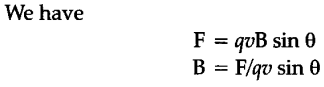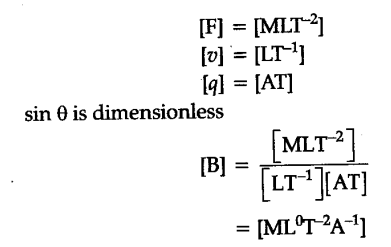# Dimensional formula of magnetic flux density (B) can be calculated from the relation F = qv Bsin , where F is force, q is charge, v is velocity and is an angle. Find [B]